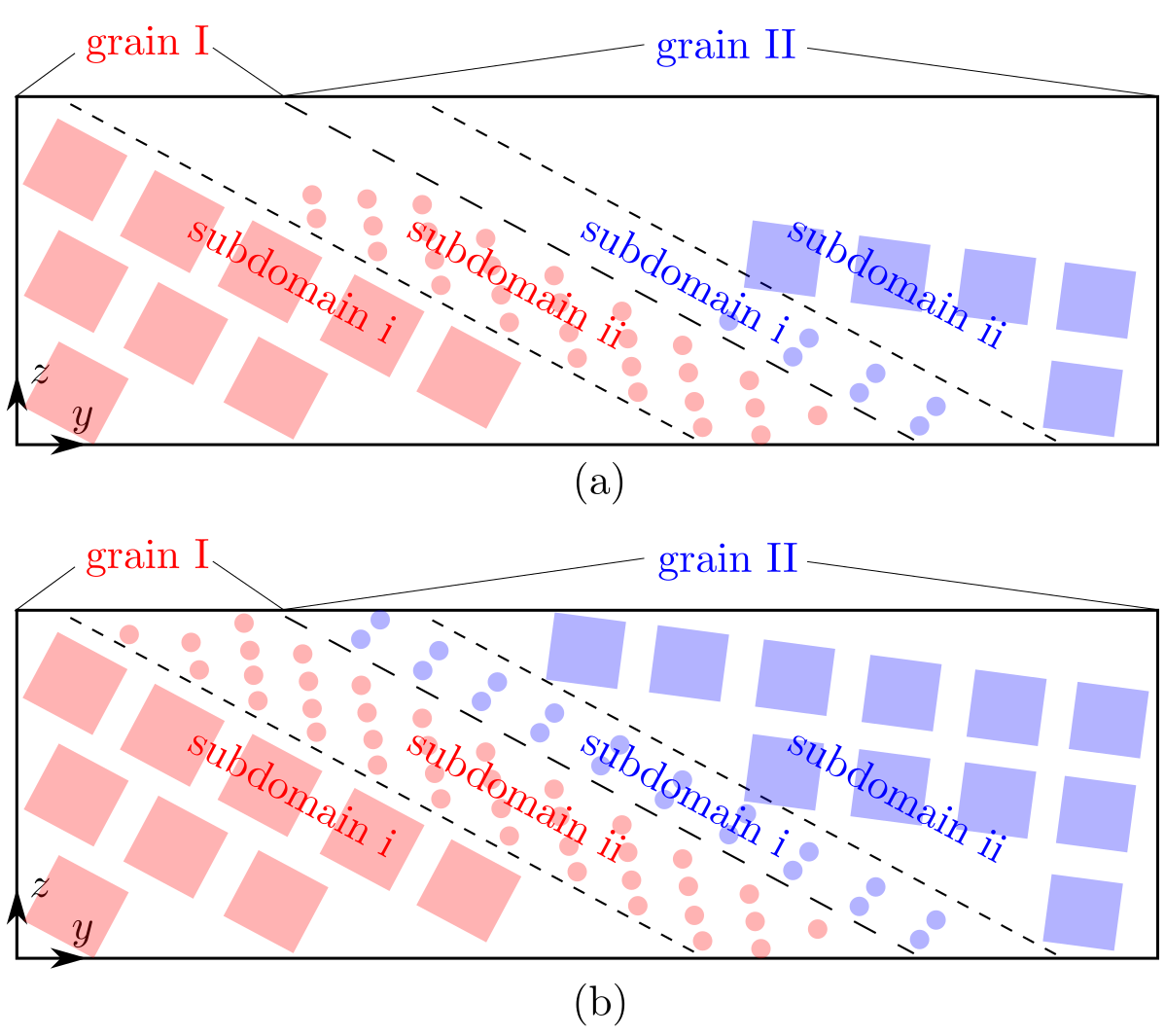# unit_num

### Syntax

unit_num {grain_id [subdomain_id x unit_num_x y unit_num_y z unit_num_z]}

• grain_id, subdomoain_id = positive integer

• unit_num_x, unit_num_y, unit_num_z = positive integer

### Examples

unit_num {1 [1 x 2 y 3 z 4]}
unit_num {1 [1 x 8 y 20 z 12] [2 x 40 y 2 z 60]} {2 [1 x 40 y 1 z 60] [2 x 8 y 25 z 12] [3 x 6 y 7 z 10]}
unit_num {1 [1 x 2 y 3 z 4]} {2 [1 x 6 y 1 z 2]} {3 [1 x 10 y 2 z 3]}


### Description

This command sets the size of each subdomain along three directions in each grain. The unit_num_x, unit_num_y, and unit_num_z are in units of the x, y, and z length of the projection of the unit (primitive unit cell in the atomistic domain or the finite element in the coarse-grained domain) on the yz, xz, and xy planes, respectively.

Similar to the unit_type command, this command consists of two loops. The outer loop, illustrated by {}, is based on grain; the inner loop, illustrated by [], is based on subdomain. Note that the curly brackets { and } as well as the square brackets [ and ] in the syntax/examples are to separate different grains and subdomains, the number of which are grain_number and subdomain_number, respectively; all brackets should not be included in preparing cac.in.

When grain_number > 1 and/or subdomain_number > 1, the size of each subdomain set directly by this command is most likely not the same, which may be problematic in some cases, e.g., in a bicrystal, as shown in Fig. (a) below, in which the subdomain i/grain I has a larger z length than the other subdomains. Since the grain stack direction is y, the size of all other subdomains along the x and z directions will be increased to match that of the subdomain i/grain I, respectively, as shown in Fig. (b) below.The three examples above correspond to the three examples in the subdomain command.

The maximum grain_id must be larger than or equal to grain_number. All information related to grain_id that is larger than grain_number is discarded. Within each grain, the maximum subdomain_id must equal the corresponding subdomain_number.

This command becomes irrelevant when boolean_restart = t, in which case there is no need for the subdomain information.

box_init.f90 and model_init.f90

None.